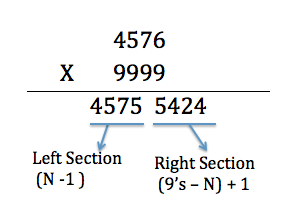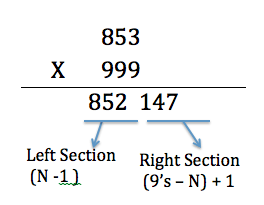# Magic Number 9 – Multiplying with 9’s

“How can I make math fun for my boys” is always an ongoing task for me as it always keeps them engaged and interested in math. They actually look forward to these games and are equally curious to understand and solve the mystery of Math.  How boring it would be if you have to do the same math worksheets every day.  How about we show them how fast they can multiply large numbers with the magic number 9. In the next couple of days, I would be sharing all the math tricks and games I use to teach my kids with my blog friends, so stay tuned.

There is a quick and fail-proof method of multiplying with 9’s. Any mathematical calculations done with magic number 9 would produce some interesting numerical patterns.

To multiply a number N with 9’s, divide the result into two sections – the left section and the right section. The left section would be ( N-1 ) and the right section would be ( 9’s – N ) +1 . The left section and right section together would be your final result.

For example,   4576 × 9999  = 45,755,424. The left section of the result would be the number 4576 – 1 = 4575 and the right section would be 9999 – 4576 = 5423 and adding 1 to it which would be 5423 +1 = 5424.Let’s try with some other number, may be  853. So, if multiplying 853 x 999 = 852,147. The left section of the result would be 853 – 1 =  852 and the right section would be 999- 853 +1 = 147.So, leave your calculators aside and have fun multiplying large numbers with 9’s in a fraction of a second

You can find some interesting patterns involving multiples of 9  here.

Want to learn more math tricks to do faster calculations, then check out these books on vedic mathematics.

If you would like to remain updated with all the new exciting posts, please consider following or liking my facebook page.

Happy Calculating !

While you are here, check out some of the latest posts

## One Reply to “Magic Number 9 – Multiplying with 9’s”

1.Fractioncalc says:

I would like to share a few tricks which i found cool.

Two digit number and its reverse always divisble by 11

The sum of a two digit number and its reverse is always divisible by 11

eg (66+66/11 =132/11=12)

89+98 /11=17 etc etc)

Magic of 37

any 3 digit number where all the digits are same is divisible 37.yes!! 333,444,555,666…all are divisible by 37
Mental Multiplication with 11

multiplication by 11,for eg 35*11=385(3(3+5)5) keep the first and the last digit same and the middle digit is the sum of the two
multiplication of numbers with same tens digit

23*27=621.

step1: 2*(2+1)=6 is the first digit.

step2: 7*3=21 second digit

multiply numbers between 11 and 19.

eg) 18*14=252.

step 1: 18+4=22

now add a zero at the end we get 220

step 2 :multiply the right most digits 8*4=32

we get 220+32=ans

The sum of 5 successive whole numbers is always divisible by 5

eg) 6+7+8+9+10=40 which is divisible by 5

The product of 3 consecutive natural numbers is always divisible by 6

eg) 6*7*8=336/6=56

The product of 3 consecutive natural numbers is always divisible by 24(if the first of the 3 natural consecutive natural numbers is even)

8*9*10=720/24=30

The last digit of the product of any 9 consecutive natural numbers is always 0

eg )(1*2*3*4*5*6*7*8*9=362880)

(as the product of any 5 consecutive natural numbers is always 0)

mentally squaring for 5

eg) 35*35=3*(3+1)…..25=1225